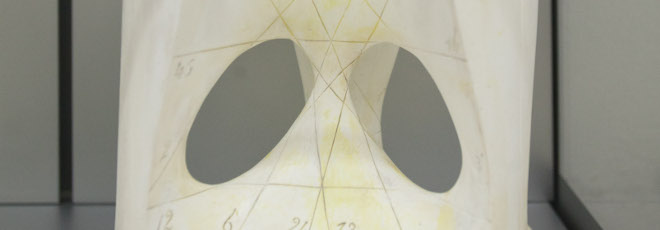## “Understanding the most likely evolution of interacting particle systems”

Venerdì 21 Febbraio 2020, ore 14:30 - Aula 1BC45 - Giovanni Conforti (CMAP, École Polytechnique)

Abstract

An old question posed by Schrodinger may be rephrased as follows: what is the most likely evolution of an interacting particle system conditionally on the observation of its initial and final configurations? It is often the case that by means Large Deviation Principle this question is rigorously formulated as a (mean field) control problem in the space of probability measures. In the first part of this talk we will see if the space of probability measures is endowed with a Riemannian metric naturally induced by the form of the rate function, the equations governing the most likely evolution take the form of Newton’s law. In some examples the Riemannian metric to be considered coincides with the Otto metric of optimal transport, but it is often the case that a different metric has to be chosen. In the second part of the talk we discuss how to use Newton’s laws to obtain convexity principles and dissipation estimates for entropy functionals along the most likely evolution. The interest of these estimates is twofold: on the one hand they imply a novel class of functional inequalities; on the other hand they allow to establish the turnpike property for the control problem defining the most likely evolution and to study its ergodic behaviour. Two concrete examples will be discussed in more detail: the exclusion process and systems of weakly interacting diffusions.

Based on joint works with A.Chiarini, I.Gentil, J.Backhoff, C.Léonard, M.Pavon and L. Tamanini